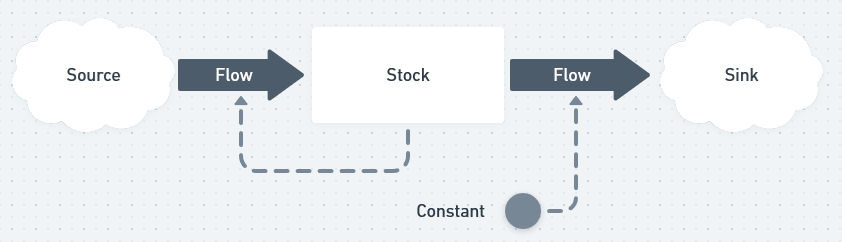# System Dynamics

## What are System Dynamics Models?

System Dynamics models represent a system as a set of stocks and the rates of flows between them. These models are powerful for capturing complex systems, and calculating their nonlinear behaviors.

Stocks represent abstract or concrete quantities present in the system, whose values change over time. For example, the number of bananas stored in a warehouse is a concrete stock, while the demand for bananas would be an abstract stock.

Flows represent rates of change for the stocks in the model. Flows are typically dependent variables, and have a rate calculated based on constants and the values of stocks in the model.Because these are the two main components of System Dynamics models, they are also referred to as Stock and Flow models. However, there are three more elements that are typically present in these models: constants, sources, and sinks.

Constants are self-explanatory. As opposed to stocks which change values, constants represent quantities which do not change. They are used as part of the calculations for flow rates, much as they are used in any mathematical equation.

Occasionally, instead of connecting two stocks, a flow will connect a source to a stock, or a stock to a sink. Sources represent quantities which are external to the system, and are effectively infinite. For instance, in a population model, a births flow could connect from a source to a newborns stock. Sinks operate in the inverse, allowing a quantity to flow out of the system. A heat transfer model might use a flow to a sink to represent heat leaving the system.## Creating a System Dynamics Model

1. Identify stocks: Begin by identifying the key stocks that will allow you to complete your analysis or project. Then continue by identifying stocks, both abstract and concrete, which are closely related to the key stocks.
2. Identify flows: Describe how the stocks in your model change over time. Flows must connect two stocks, or between a stock and a source or sink.
3. Define equations for your flows: The rate for the flows must be based on a mathematical combination of constants and/or the values of stocks.
4. Capture constants: Define the constants you used in the definitions of the flows, and provide them with numeric values.

## Why use System Dynamics Models?

System dynamics models are excellent for understanding complex systems that change over time. When a system of interest is exhibiting nonlinear behavior, a well-designed system dynamics model can often reproduce the behavior.

Creating a SD representation of a system can function as a digital twin for real-world operations, allowing you to simulate many different scenarios and exogenous shocks. Assembling, testing, and refining an SD model will also give modelers insight into the structure of a system, discovering unintuitive feedback loops and hidden delays. Organizations can capitalize on these virtual, low-cost insights to make real-world cost-saving and productivity improvements.

Read more about building a system dynamics model in HASH >

##### Quick Jump
What are System Dynamics Models?
Creating a System Dynamics Model
Why use System Dynamics Models?